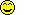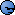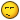# Cp Doen't Solve This Simple Equation.

6 replies to this topic

### #1Serj

Serj

Newbie

•• Members
•• 15 posts

Posted 13 June 2007 - 01:31 PM

I did a solve(0.5 * 1E-4 * 32 * (x-0.7)^2 -4E-4 = 0, x) and it gave me these solutions:
x=0.9 or x=1.9 . Which are completely wrong and really screwed me because this was during an exame, I only have this calc over a month and it's really pissing me off. Any comments?

Oh and I have os 3.1 ..

### #2Serj

Serj

Newbie

•• Members
•• 15 posts

Posted 13 June 2007 - 01:41 PM

I discovered something new, if i do the solve( in Standard it gives me a solution that is 6/5, which is 1.2 and is the right solution.

Is this a bug, if so its really stupid, like the not being able to do comples exponentials in degrees. Very bad Casio### #3vanhoa

vanhoa

Casio Overlord

•• Members
•• 854 posts
• Gender:Male
• Location:Vietnam

• Calculators:
AFX 2.0, CP 300, CP 330, nSpire, TI 89, FX 5800

Posted 13 June 2007 - 01:49 PM

I found nothing wrong...

(CPM OS 3.0)### #4Serj

Serj

Newbie

•• Members
•• 15 posts

Posted 13 June 2007 - 02:14 PM

Doing it that way also gives me the right answer.. but doing exactly solve(0.5 * 1E-4 * 32 * (x-0.7)^2 -4E-4 = 0, x)
it still gives the error. Vanhoa, it this normal?

### #5vanhoa

vanhoa

Casio Overlord

•• Members
•• 854 posts
• Gender:Male
• Location:Vietnam

• Calculators:
AFX 2.0, CP 300, CP 330, nSpire, TI 89, FX 5800

Posted 13 June 2007 - 03:30 PM

Yes... This is not normal.

0.9 and 1.9 are roots of (x-7/5)^2/625-1/2500

I dont know why 7/10 can be changed to 7/5...

### #6vanhoa

vanhoa

Casio Overlord

•• Members
•• 854 posts
• Gender:Male
• Location:Vietnam

• Calculators:
AFX 2.0, CP 300, CP 330, nSpire, TI 89, FX 5800

Posted 13 June 2007 - 03:59 PM

Let's take a look:

(CPM OS 3.0)
This is calculated in both decimal and standard mode:

solve( a * ( x - b ) ^ 2 = c )

{x=b-(a*c)^0.5/a,x=b+(a*c)^0.5/a}

And then:
This is calculated in
standard mode:

solve( a * ( x - b ) ^ 2 = c | b = 0.1 )
{x=(-(a*c)^0.5)/a+0.1,x=(a*c)^0.5/a+0.1}

This is calculated in decimal mode:

solve( a * ( x - b ) ^ 2 = c | b = 0.1 )
{x=(-(a*c)^0.5)/a+0.2,x=(a*c)^0.5/a+0.2}

But:
This is calculated in standard mode:

solve( a * ( x - b ) ^ 2 = c | b = 1 )
{x=(-(a*c)^0.5)/a+1,x=(a*c)^0.5/a+1}

This is calculated in decimal mode:

solve( a * ( x - b ) ^ 2 = c | b = 1 )
{x=(-(a*c)^0.5)/a+1,x=(a*c)^0.5/a+1}

=> if
b is decimal, it is multiplied by 2(Only on OS 3!)

### #7Serj

Serj

Newbie

•• Members
•• 15 posts

Posted 13 June 2007 - 09:01 PM

Damn Vanhoa.. you really must have a programmers brain because you decomposed that to see where the algorithm was wrong. It is the most straight forward one, but maybe it makes an error while rewriting that equation. Anyway one more for the bug list.

#### 0 user(s) are reading this topic

0 members, 0 guests, 0 anonymous users